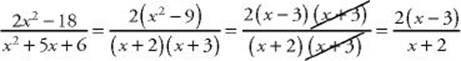﻿ ﻿ALGEBRAIC FRACTIONS - Polynomials - ALGEBRA - SAT SUBJECT TEST MATH LEVEL 1

## ALGEBRA## CHAPTER 5 Polynomials### ALGEBRAIC FRACTIONS

Although the coefficient of any term in a polynomial can be a fraction, such asthe variable itself cannot be in the denominator. Expressions such aswhich do have variables in their denominators, are called algebraic fractions. You will have no trouble manipulating algebraic fractions if you treat them as regular fractions, using all the standard rules for adding, subtracting, multiplying, and dividing.

Whenever you have to simplify an algebraic fraction, factor the numerator and denominator, and divide out any common factors.

TIPAll the rules for numerical fractions apply to algebraic fractions.

EXAMPLE 9: Simplify.Note thatis an identity. For every real number (except –2 and –3, for which the original fraction is undefined), the expressions have the same value. For example, when x = 8:Similarly, when x = 3, both expressions equal 0, and when x = –4, both expressions equal 7.

﻿